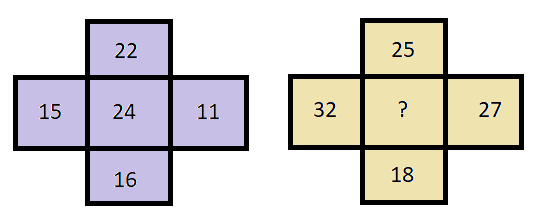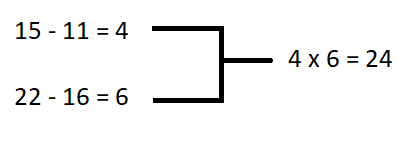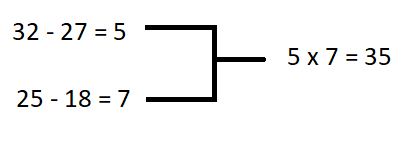# Question of the day-Logical Reasoning:17-June-2019

2019-06-17 | Team PendulumEdu

In the given question which number among the given alternatives will replace the question mark?A. 35

B. 26

C. 45

D. 38

Solution

Considering the first figure we can deduce the logic asSimilarlyAfter looking at the logic applied in the purple figure, we can apply the same logic in the yellow figure and get the answer. In such type of questions, try to do subtraction, addition, multiplication and division of diagonal and opposite elements, and try to establish a connection.

Hence, (A) is the correct answer.0